# Radioactive Decay, Formula, Types and Examples

Rashid Nuzha, Nissa Garcia
• Author
Rashid Nuzha

In 2021, Rashid earned a Postgraduate Certificate in Professional Studies in Education (PGCPSE) from The Open University-United Kingdom. In 2019, he obtained ITTT 120-hour TEFL Certificate. Rashid has held a BSc in Physics and Mathematics since 2005. Also, Rashid has 7-years of experience in educational leadership and management.

• Instructor
Nissa Garcia

Nissa has a masters degree in chemistry and has taught high school science and college level chemistry.

Learn the radioactive decay definition. Know the radioactive decay formula. Explore the differences between alpha decay, beta decay, and gamma emission. Updated: 03/26/2022

Show

To understand radioactive decay definition, recall that nuclide is a nucleus with a specific neutron number N and atomic number or number of protons Z, therefore, a specific mass number A=N+Z. Nuclides can be stable and unstable. So, to know what is radioactive decay, it is the case when the unstable nuclides emit particles or electromagnetic radiations and turn to other nuclides. In some nuclides, the continuous process of radioactive decaying takes less than a microsecond, but in some other nuclides, it may continue to radioactive decay for billions of years. Keep in mind that an unstable radioactive nuclide loses its mass while emitting particles.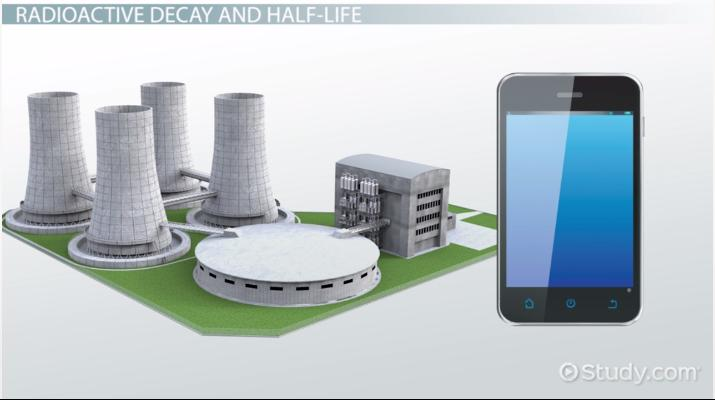An error occurred trying to load this video.

Try refreshing the page, or contact customer support.

Coming up next: What Are Radioactive Substances? - Examples & Uses

### You're on a roll. Keep up the good work!

Replay
Your next lesson will play in 10 seconds
• 1:55 A Review of Chemical Symbols
• 2:33 Alpha Decay
• 3:30 Beta Decay
• 5:15 Gamma Emission
• 6:00 Lesson Summary
Save Save

Want to watch this again later?

Timeline
Autoplay
Autoplay
Speed Speed

The famous physicists Marie Curie and Ernest Rutherford, among many others, discovered that radioactive decay results in positive and negative particles and neutral rays. Due to the different penetration characteristics, the three results were given the names alpha decay, beta decay, and gamma decay. So, when an unstable nuclide starts emitting radioactive materials, it is called the parent nucleus, and the result new nuclide is called the daughter nucleus. Every nuclide can be represented as {eq}_{Z}^{A}\textrm{X} {/eq} where the symbol of any nuclide replaces X, A is the atomic mass, and Z is the number of protons. Keep in mind that A=Z+N, where N is the number of neutrons.

Due to mass-energy conservation laws during decay, the unstable parent and the result daughter nuclides are related by their atomic mass numbers A and atomic numbers Z. So, The parent's atomic number must be equal to the daughter's atomic number plus the emitted particle atomic number. Also, the parent's atomic mass number must equal the daughter's atomic mass number plus the emitted particle atomic mass number.### Alpha Decay

The helium nucleus {eq}_{2}^{4}\textrm{He} {/eq} is called an alpha particle which is made of two protons and two neutrons. In alpha decay or alpha emission, the unstable parent nuclide emits an alpha particle.

An example of alpha emission is the radioactive Radium nuclide Ra-226 turning to Radon Rn-222 by emitting an alpha particle:

{eq}_{88}^{226}\textrm{Ra}\rightarrow _{86}^{222}\textrm{Rn}+_{2}^{4}\textrm{He} {/eq}

The atomic mass and numbers are balanced in this radioactive process. Notice that, {eq}A_{Parent}=A_{Daughter}+A_{Particle} {/eq} or {eq}226=222+4 {/eq}. Also, {eq}Z_{Parent}=Z_{Daughter}+Z_{Particle} {/eq} or {eq}88=86+2 {/eq}

So, in alpha decay, the atomic mass number A is reduced by four, and the atomic number Z is reduced by 2.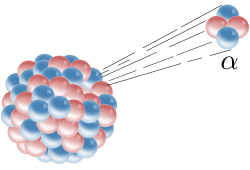### Beta Decay

There are two types of beta decay or beta emission: beta-minus decay and beta-plus or positron decay. Beta-minus {eq}\beta ^{-} {/eq} particle is an electron {eq}_{-1}^{0}\textrm{e} {/eq}. Beta-plus or positron {eq}\beta ^{+} {/eq} is the opposite particle to an electron in terms of the charge or the proton.

Example 1:

An example of beta-minus decay or emission is the radioactive Cobalt nuclide Co-40 turning to Nickle Ni-40 by emitting an electron:

{eq}_{27}^{40}\textrm{Co}\rightarrow _{28}^{40}\textrm{Ni}+\beta^{-1} {/eq}

The atomic mass and numbers are balanced in this radioactive process. Notice that, {eq}A_{Parent}=A_{Daughter}+A_{Particle} {/eq} or {eq}40=40+0 {/eq}. Also, {eq}Z_{Parent}=Z_{Daughter}+Z_{Particle} {/eq} or {eq}27=28+(-1) {/eq}

So, in beta-minus decay, the atomic mass number A stays the same, and the atomic number Z increases by 1. The daughter nucleus in beta-minus decay has a larger mass than the parent nucleus.

Example 2:

An example of beta-plus decay or emission is the radioactive Carbon nuclide C-11 turning to Boron B-11 by emitting a positron:

{eq}_{6}^{11}\textrm{C}\rightarrow _{5}^{11}\textrm{B}+\beta^{+1} {/eq}

The atomic mass and numbers are balanced in this radioactive process. Notice that, {eq}A_{Parent}=A_{Daughter}+A_{Particle} {/eq} or {eq}11=11+0 {/eq}. Also, {eq}Z_{Parent}=Z_{Daughter}+Z_{Particle} {/eq} or {eq}6=5+1 {/eq}

So, in beta-plus or positron decay, the atomic mass number A stays the same, and the atomic number Z is decreased by 1. The daughter nucleus in positron decay has a smaller mass than the parent nucleus.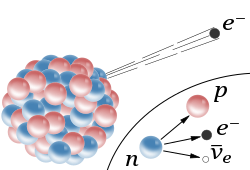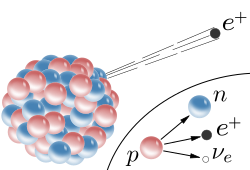### Gamma Emission

Recall that a photon is a sack or bundle of energy with no mass and no charge, which makes up electromagnetic waves. For example, light is believed to be dual-natured, one of which is looked at as photon waves. Gamma rays are another name for photons. In gamma {eq}\gamma {/eq} emission or decay energy is released as gamma-ray photons or electromagnetic waves when an excited nuclide moves to a more stable or less excited state. In general, other decays involve releasing energy or emitting gamma rays.

• Notice that the major difference between alpha, beta-minus, beta-plus forms of decay and gamma decay is that in gamma decay, the element does not change.

Example:

An example of gamma decay or emission is the excited radioactive Protactinium nuclide Pa-234 turning to less excited Protactinium Pa-234 by emitting a gamma-ray photon:

{eq}_{}^{234}\textrm{Pa}{}^{*}\rightarrow _{}^{234}\textrm{Pa}+\gamma {/eq}

Notice that star (*) refers to the excited state. Also, both parent and daughter nuclei are the same element since gamma rays have no atomic mass or atomic number.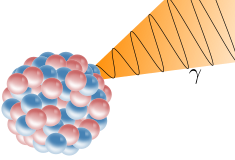The radioactive decay formula is given by:

• {eq}N(t)=N(0)*e^{-\lambda *t} {/eq}

where N(t) is the number of remaining nuclei at time t measured in seconds (S), N(0) is the initial number of nuclei at time t=0, and {eq}\lambda {/eq} is the decay constant that differs for different nuclides and is measured by 1/seconds or 1/S (S^{-1})

The radioactive decay equation shows that the number of nuclei is reduced exponentially by time so that the formula can be written as {eq}\frac {N(t)}{N(0)}=e^{-\lambda *t} {/eq}

To know how to calculate radioactive decay, use the known values of the initial amount to nuclei at t=0 and the decay constant of the unstable element, then at any time t by substituting all values in the formula, one can find out how many of the element's nuclei are left N(t).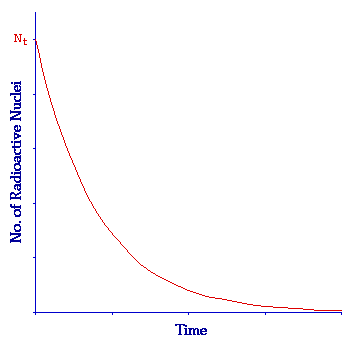### Decay Constant Formula

In particle physics, lifetime is the life of an unstable particle or nucleus while it is still active. In general, lifetime is referred to as {eq}T_{mean} {/eq} which is equal to {eq}\frac {1}{ \lambda} {/eq} or the reciprocal of the decay constant.

So, lifetime formula is: {eq}T_{mean}=\frac {1}{ \lambda} {/eq}, or decay constant formula is: {eq}\lambda=\frac {1}{ T_{mean}} {/eq}

### Half Life Radioactive Decay Formula

A decaying radioactive material follows the formula: {eq}-\frac{\mathrm{d} N(t)}{\mathrm{d} t}=\lambda *N(t) {/eq} where the minus sign refers to the decaying or decrease in the number of nuclei N(t) over time. So, the radioactive mass is reduced exponentially following the formula: {eq}N(t)=N(0)*e^{-\lambda *t} {/eq}.

Consider the case that the amount of radioactive material is reduced to half its initial amount. For example, consider at time t where the current N(t)=25g, as half the initial N(0)=50g. The time that has passed till half as much remains of the radioactive material is called half-life decay.

Now, substituting {eq}N(t)=(\frac {1}{2})*N(0) {/eq} or {eq}\frac {N(t)}{N(0)}={1/2} {/eq} in the exponential formula of N(t) gives: {eq}{1/2}=e^{-\lambda *t} {/eq}. Simplifying the formula to get the time t in terms of the decay constant {eq}\lambda {/eq} gives: {eq}T_{1/2}=\frac {ln2}{\lambda}=\frac {0.693}{\lambda} {/eq}.

{eq}T_{1/2} {/eq} is called the half-life decaying time it took the decaying radioactive material to lose half its initial mass.

So, half-life radioactive decay formula is given by:

• {eq}T_{1/2}=\frac {0.693}{\lambda} {/eq}

To unlock this lesson you must be a Study.com Member.

#### What does radioactive decay mean?

Radioactive decay means that an unstable nuclide emits particles or rays and turns into another nuclide. The unstable nuclide is called the parent nucleus, and the result of radioactivity is called the daughter nucleus. Depending on the nuclide, radioactive decay may last from less than a microsecond to billions of years.

#### What is the formula for calculating half-life?

Half-life is the time it takes an initial amount of radioactive material to be reduced to its half. The half-life formula is given as:

T_{1/2}=\frac {0.693}{\lambda}

where \lambda is the decay constant

### Register to view this lesson

Are you a student or a teacher?